# Input-Output Quiz For IBPS PO: Part 7

Directions (Q. 1-5): Study the following information carefully and answer the questions given below:
A number arrangement machine when given an input line of numbers rearranges them following a particular rule in each step. The following is an illustration of input and various steps of rearrangement.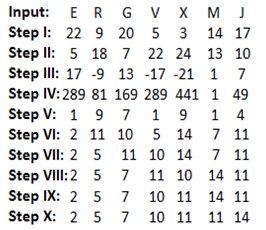And Step X is the last step of the rearrangement as the desired arrangement is obtained. As per rules followed in the above steps, find out in each of the questions the appropriate step for the given input.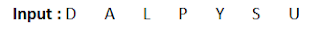Q1. Which of the following is fourth from the left end of Step VI in the above arrangement?
A. 12
B. 11
C. 10
D. 9
E. 15

Q2. How many steps are needed to complete this arrangement?
A. V
B. VII
C. IX
D. X
E. VIII

Q3. Which of the following represents the first two and the last two elements in the last step?
A. 2, 6, 12, 11
B. 2, 6, 12, 16
C. 3, 5, 12, 16
D. 3, 5, 12, 15
E. None of these

Q4. What is the position of ‘11’ in the sixth step of the output?
A. 2nd from the left end
B. 2nd from the right end
C. 3rd from the right end
D. 4th from the left end
E. None of these

Q5. How many elements are there between 2 and 12 in step VII?
A. 5
B. 6
C. 4
D. 2
E. 0

#### 1. Ans. B.

Solution:#### 2. Ans. E.

Solution: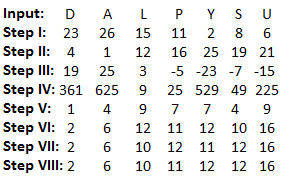#### 3. Ans. B.

Solution: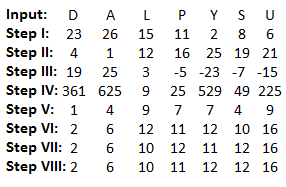#### 4. Ans. D.

Solution: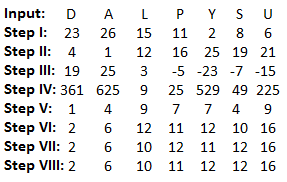#### 5. Ans. D.

Solution: